##### Search### SECTION A (25 Marks)

Answer all the questions in the spaces provided.
1.

The figure below shows a measuring cylinder which contains water initially at a level A. A spherical solid of mass 11g is immersed in the water, the level rises to B.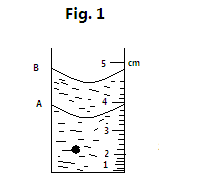Determine the diameter of the spherical ball

2 marks

2.

Explain the reason why a dropping dust particle in a still room does not trace a straight vertical path

1 marks

3.

Two candles, a short and long candle were lit and then covered with a tall bell jar as shown in figure 2 below. State and explain which of the candles would go off first.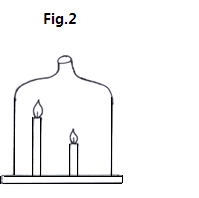2 marks

4.

The figure 3 below shows a beaker containing water placed on a flat bench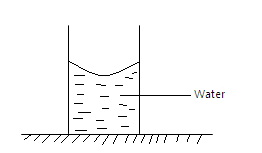State and explain the changes in stability of the beaker when the water freezes to ice

3 marks

5.

Figure 4 below shows a solid sphere moving on a platform 5cm above the ground. It rolls down a curved frictionless path in a point 0.5m above the ground.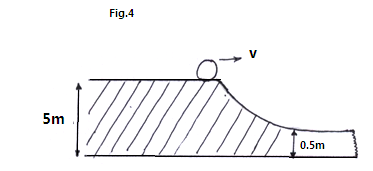Calculate its velocity at the lower point

3 marks

6.

Two table tennis balls hang at the same level suspended from a thread a short distance apart. A stream of air is blown between the balls. Explain what happens to the balls

2 marks

7.

Figure 5 shows a bubble of soap blown to the wider end of a funnel.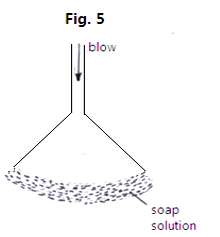When the top is left open the bubbles flatten to a film which rises up the funnel. Explain observation.

3 marks

8.

State two advantages of a force pump over lift pump

2 marks

9.

Using the kinetic theory of gases, explain why air inside a tyre exerts pressure on the walls of the tyre

2 marks

10.

When ice is heated, the temperature remains constant until all ice is melted. Explain this observation.

1 marks

11.

Figure 6 below shows two strings A and B made of the same material. The thickness of the wires and lengths are the same. Which of them is likely to stretch out first when hooked masses are loaded to their lowest end?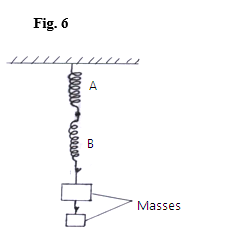2 marks

12.

A uniform half meter rule is supported by force of 3N and 2N as shown in figure 7 below.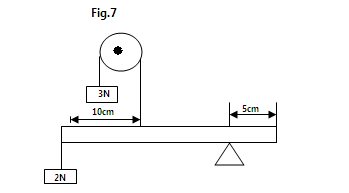Determine the weight of the half meter rule

2 marks

13.

A high jumper usually lands on thick soft mattress. Explain how the mattress helps in reducing the force of impact

1 marks

14.

The figure 8 shows a sketch of a displacement-time graph.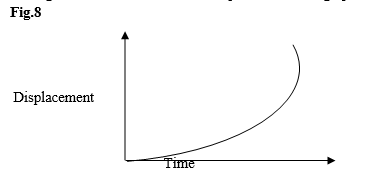Describe the motion as displayed by the graph

2 marks

### SECTION B (55 Marks)

Answer all the questions in this section in the spaces provided.
15.

. a) State any one form of energy

b) An electric crane lifts a load of 2000kg through a vertical distance of 3.0m in 6 seconds Determine:

i) The work done

ii) The power developed by the crane

The efficiency of the crane if it is operated by an electric motor rated 12.5kw. (2mrks) __________________________________________________________________ __________________________________________________________________ __________________________________________________________________ _______________________________________
c) A bob of mass 20kg is suspended using a string 4m long from a support and swung
through a vertical height of 0.9m as shown in figure 9 below.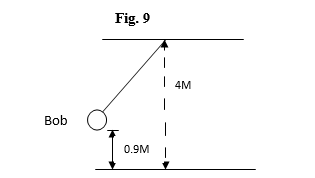Determine;
i) The potential energy of the bob at its position shown __________________________________________________________________ __________________________________________________________________ __________________________________________________________________

_______________________________________

ii) The speed of the bob when passing through the lowest point during the swing

11 marks

16.

a) A car is said to be decelerating. Explain ________________________________________________________________________ ________________________________________________________________________ ________________________________________________________________________ __________________________________________

b) The figure 10 below shows an idealized displacement-time graphs for the journey of a train a long a straight horizontal track from the moment when it passes a point A on the track.

GRAPH

Use the grid below to plot a velocity-time graph of the journey shown in figure 10. Clearly show your workings.

GRAPH

c) A space shuttle is in orbit round the earth at a certain height.
i. What keeps it in the orbit?

____________________________________________________________ ____________ ____________________________________________________________ ____________ ____________________________________________________________ ____________
ii. In which direction is it accelerating at each point in its orbit

iii. A girl ties a stone of mass 250kg with a piece of thread. Holding the other end of the thread at 45cm in length, she makes the stone revolve in a circle in a vertical plane at a constant speed. If the stone is making 3 revolutions, per second calculate;

a) The angular velocity

______________________________________________________ ______________________________________________________ ______________________________________________________ ______________________________________________________ ______________________________________________________ ______________________________________________________ _

b) Tension in the string when the stone is vertically above the girls hand ______________________________________________________

8 marks

17.

a) In an experiment an aluminum block of mass 2kg was heated using immersion heater as shown below in figure II.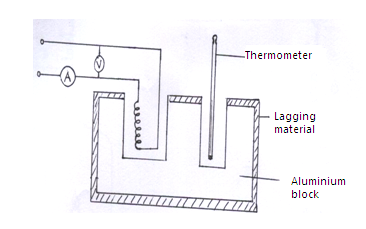The temperature of the block was recorded every minute for exactly five minutes and the heater was switched off. A graph of temperature in 0C against time in minutes for the experiment as shown below.

GRAPH

Study the graph and answer the following questions;
i. What was the room temperature

ii. Suggest why the reading of the thermometer rose relatively slowly between point A and B ________________________

8 marks

18.

a) State the law of conservation of linear momentum.

b) A metal block A of mass 40kg requires a horizontal force of 100N to drag it with uniform velocity a long a horizontal surface as shown in the figure 12 below.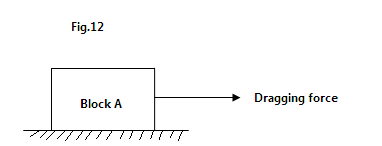i. calculate the coefficient of friction

Another block B of mass 50kg requires a force of 125N to drag it along the same surface. The two blocks A and B are now connected together with a tow-bar and a dragging force of 275N applied to pull them along the same surface as shown below in figure 13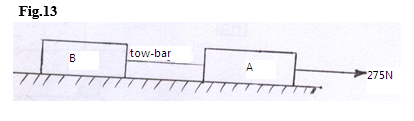Determine;
i. The acceleration of the system

__________________________________________________________________ __________________________________________________________________ __________________________________________________________________

_______________________________________

ii. The tension of the tow-bar

__________________________________________________________________

c) A fisherman in Nduru beach wanted to jump out of his boat towards the off shore.

Unfortunately he landed in water. Explain why he landed in water.

10 marks

19.

a) The level of water in a measuring cylinder rises from the 50cm3 mark to 55.7cm3 marks when a metal block weighing 45g is submerged in the water in the cylinder

. i. Calculate the density of the metal block

__________________________________________________________________ __________________________________________________________________ __________________________________________________________________ __________________________________________________________________

____________________________________________________

ii. State two differences between density and relative density

b) In an experiment to determine the density of solid S which is not soluble in water. A student obtained the following;
- Mass of empty density bottle= 20g
- Mass of density bottle when full of water =45g
- Mass of density bottle with small quantity of solid S =152g
- Mass of density bottle with small amount of solids S and topped up with water =167g
Given that the density of water is 1g/cm3,
Find;
i. The volume of the density bottle

_________________________________________________________________

ii. The mass of the solid S

iii. The volume of the solid S _________________________________________________________________

10 marks

Back Top